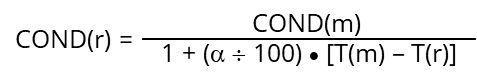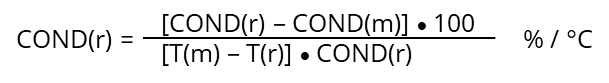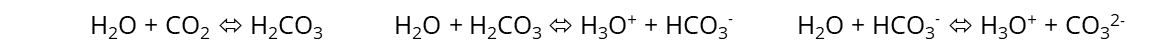# Introduction

With the electrolysis of a salt solution, a voltage is applied to two electrodes, one becoming the positive anode, the other the negative cathode. With the ions in solution, a migration starts for anions (negatively charged ions like Cl-) to the anode and for cations (positively charged ions like Cu2+) to the cathode. So, the ions transport the electrical charge through the solution and are electrochemically modified, i.e. oxidized (anions) or reduced (cations).

Similar is the function of a battery, where e.g., a copper electrode is in a copper sulfate solution and zinc electrode is in a zinc sulfate solution are separated but in contact through a diaphragm. With differences in the solution’s concentrations a migration of ions develops and with different normal potentials of the metals involved a voltage can be measured (so called “Daniel Element”).

The specific electrical conductivity σ [S/m] is a physical parameter describing the ability of a substance (liquids, metals, gases) to conduct electrical current. In liquids, the electrical conductivity is caused by positive and negative charged ions that are able to interact with an applied electrical field. In general, the conductivity is dependent on the concentration and type of ions, and the temperature.

There are two basic principles for measuring conductivity, inductive (non-contacting) and contacting.

Inductive sensors are using two electromagnetic coils usually encased in a polymer ring. An alternating voltage is applied to the driving coil, which induces a voltage in the receiving coil. The induced current is influenced by the conductance of the solution. Due to the sealed casing, inductive sensors can be used in aggressive environments, but they are not suited for conductivities below 15 µS/cm.

Contacting sensors consist of conducting electrodes (e.g., metals like platinum, gold, steel, titanium, and non-metal graphite) being in direct contact with the medium. The primary parameters measured are voltage and the applied current. The interdependence of both parameters, described by Ohm’s law, allows for the calculation of a resistance R (measured in Ohm [Ω]) or the inverse – the conductance G (measured in Siemens [S]). To avoid electrochemical redox reactions and polarization effects at the electrodes, alternating currents with frequencies between < 100 Hz up to 50 kHz are applied. The measured current/voltage ratio depends on the properties of the solution and of the sensor, i.e. the volume (length and area) through which the current flows. This current path is defined by the sensor geometry and expressed in the so called cell constant K, which by definition is the ratio of the electrode distance [cm] and area [cm²]. Except in norm and research institutes, like DIN, ISO, NIST, PTB etc.(*), where the cell constant is calculated from dimensional measurements, in practice, the cell constant is determined using a solution of known conductivity. The cell constant is the ratio of the known conductivity [S/cm] to the measured conductance [S].

Multiplying the measured conductance by the cell constant corrects for the individual properties of the sensor. The result is the conductivity σ, which only depends on properties of the solutionBasic parameters and their relationships

 Voltage U [V] Current I [A] Resistance R [Ω] Conductance G [S] = 1/R Ohm’s law R = U / I -> G = I / U Specific resistivity ρ: [Ωm] Specific conductivity σ: [S/m] (σ = 1/ ρ) Electrode distance d [cm] Electrodes area A [cm2] Cell constant K = d/A [cm-1]

Typically, the cell constant is determined in the factory and entered into the associated conductivity meter or converter. To achieve the highest possible accuracy it is recommended to calibrate the system when installed in the process using a calibration solution having a nominal conductivity within the desired measurement range and temperature.

## The conductivity scale

Between the standard unit “S Siemens” (100) and the mostly used unit “µS micro Siemens” (10-6) there is a difference of 106 = 100.000. If a conductivity sensor measures from µS up to mS (milli Siemens 10-3) up to S, it is obvious that the sensor performance cannot be the same over the whole range. That is why conductivity sensors have their accuracy linked to the measuring range.

 Typical conductivity values at 25°C (77°F) High purity water 0.05 μS/cm Tap water 500 μS/cm Acetic acid 10 % 1.76 mS/cm NaOH 10 % 353 mS/cm

## Influencing Factors

The accuracy of conductivity measurements is influenced by many factors most of which have been compensated for during the evolution of conductivity sensors.

#### Cable resistance and capacitance

Cable resistance is only an issue when working with two-pole sensor since in a four pole or six electrode sensor there is hardly any current flow via the voltage measuring electrodes that would be influenced by cable resistance. Cable capacitance needs to be taken into consideration even when working with four pole sensor. Especially at low conductivities the influence of the cable becomes relatively big and needs to be minimized e.g., by lowering the frequency of the applied current. Typical ranges for cable resistance and capacitance are 0…2000 Ω and 0…2000 pF.

#### Temperature

Since conductivity in liquids is usually increasing with increasing temperatures, all conductivity meters are equipped with a temperature compensation function. Conductivity standards available for a wide range of conductivities always come with a table showing the conductivity of the standard at several temperatures, see DIN EN 27888. Because of the strong dependency of electrical conductivity from temperature any conductivity sensor should have an integrated temperature sensor.

#### Temperature correction

While standard temperatures like 20 or 25 °C (68 or 77 °F) are used for calibrations with conductivity standards, the measurement of sample conductivity usually is performed at different temperatures. In order to compare actual and historic measurements, or comparing conductivities of different samples, it is common practice to recalculate the measured conductivity to a standard temperature, such as 20 or 25 °C – or none. Most conductivity meters and converters offer this function, where the user can select the required standard temperature. The measurements are then stored as temperature corrected values”.

However, this temperature standardization can be made in a limited temperature range, near the calibration and sample measurement temperature. A rule of thumb is calibration temperature +/- 5°C can be used for correction. Beyond this range a sample temperature adjustment (cooling / heating) or a new calibration must be performed – to keep the accurate measurement result.

#### Temperature compensation

Aqueous solutions change the conductivity with temperature. Most sample behavior can be characterized by a linear “temperature coefficient” α (%/°C). This coefficient has to be entered manually into the converter setup, where the temperature correction and compensation is calculated following the formula:COND(m) conductivity measured at measurement temperature T(m) COND(r) conductivity calculated at reference temperature T(r) T(m) measurement temperature (°C) T(r) reference temperature (°C) α temperature coefficient (%/°C)

If the temperature coefficient of the specific sample is not known, it can be determined experimentally measuring the sample at reference temperature T(r) = 25°C and at second temperature T(m) like 15 or 35 °C (difference of 10°C or more).For “natural waters” (6…100 mS/m) those “non-linear” temperature coefficients are listed in DIN EN 27888. In case existing conductivity results have been measured at 20°C they can be recalculated to 25°C by multiplying with the factor 1.116.

#### Polarization

Applying an electrical current to electrodes in solution causes the formation of a layer of counter ions close at the electrodes surface resulting in an additional capacitive resistance. The influence of this capacitive resistance is minimized by applying an alternating current (AC) avoiding the formation of ion layers. Higher AC frequencies are applied when measuring high conductivities, where the polarization resistance is big compared to the solution resistance. At low conductivities low AC frequencies are applied, which are less influenced by the cable capacity. When using a four pole cell the polarization resistance has hardly any effect on the measurement.

#### Geometry and cell constant

The quotient d/A [cm-1] is traditionally known as cell-constant (K) describing the area of the electrical field used to determine the conductivity. With the electrode arrangements of modern conductivity sensors the cell constant can be calculated by given dimensions (theoretical cell constant), but the real electrode area and surface structure adds to a “real cell constant”, which must be determined indirectly by a calibration in conductivity standard solutions. The measurement cell volume around the conductivity cell influences the cell constant as well. That is why a calibration of the completely installed system must be performed, when a new conductivity sensor is used. One factor influencing the properties of the electrical field is the ion concentration in the solution. This is why most modern sensor-transmitter systems work with editable cell constants to adjust for a wide conductivity range (µS up to S), using conductivity sensors with different cell constants or based on range specific calibrations.

#### Durability / shelf-life of conductivity standard solutions

The commercially available conductivity standard solutions cover a wide range of conductivities. While solutions of higher conductivities > 1000 mS can compensate for little contaminations over time, lower conductivities can change their nominal values quickly, e.g., if exposed to ambient air. Therefore it is good laboratory and manufacturing practice (GLP / GMP) to carefully check any conductivity standard before use. Old solutions and those opened many times for calibrations should be replaced by new standard solutions to maintain a constant level of accurate calibrations.

#### Accuracy of conductivity standard solutions

As stated before, the shelf-life is critical to conductivity standards, especially with low nominal values in µS range. This is why norms like DIN EN 27888 describe conductivity standards and their temperature behavior. Potassium chloride solutions of 1, 0.1 and 0.01 molar solutions are commonly used, having conductivities of 111.8, 12.88, and 1.408 mS/cm at 25°C. Those high concentrated KCl salt solutions can be produced with a final accuracy of +/-0.5 %. However, low conductivity standards like 147 or 25 µS/cm solutions can go up to 5% accuracy. The accuracy directly goes into the uncertainty calculation of the sample measurement. A higher accuracy better than that of the standard cannot be achieved in sample measurements.

#### CO2 effect

Electrical conductivity in solutions is strongly influenced by any additional ions and dissolved gases. Ambient air consists of nitrogen (N2), oxygen (O2), carbon dioxide (CO2) and other nobel gases in lower concentrations. While N2 and O2 do not “dissolve” chemically as ions in aqueous solutions, CO2 does. It forms the carbonic acid H2CO3, which dissociates into ions:The contact between low conductivity standards and ambient air must be avoided, because the CO2 adds ions to the standard solutions and changes the nominal value. Experiments have shown that ultrapure water starting with 0.05 µS/cm pouring into a glass beaker raises up to 1 µS/cm within seconds and moves even higher over time. The equilibrium between CO2 in air and in the aqueous solution is the driving force. This is why any measurement below 50 µS/cm must be made in sealed measurement cells and below 10 µS/cm an inert protection gas, like nitrogen N2, must be used to keep any air out of the solution or measurement cell (purge). With low conductivities it is even better to measure the sample in a sealed “flow-through” cell.

#### Stirring the sample

If not measuring the sample inline or in a flow, it is recommended to have a slight stirring (e.g., by a magnetic stirrer), but don’t create a vortex introducing air into the solution (adding CO2 !).

#### Verification and calibration of conductivity sensors

After using the sensor, the surface may suffer from chemical and mechanical treatment by the sample being measured. This typically leads to a change of the surface of the pole material and therefore to a change of the cell constant (see above). To get the actual, real behavior of the conductivity cell it is recommended to regularly calibrate the sensor. Norms like ISO 27888 describe conductivity standard solutions, like potassium chloride solutions (KCl) with 0.001, 0.01 and 1 mol/L. Those solutions have the following conductivities at 25°C:

 0.001 mol/l KCl 14.7 mS/m 147 µS/cm 0.01 mol/l KCl 141 mS/m 1408 µS/cm 0.1 mol/l KCl 1290 mS/m 12.88 mS/cm

There are conductivity standard solutions available with 5 µS/cm or 1 µS/cm. Because of the risk of contamination with CO2 from ambient air entering the bottle when opening, the certified conductivity value will change immediately. That is why those solutions can be used in closed systems only and adding inert gas (e.g., N2) if possible.

Ultrapure water comes with 0.05 µS/cm from the water purification cycle. This can be used to check the zero point of the sensor, if the UPW is used in closed systems only and adding inert gas (e.g., N2) if possible. Because of the risk of undefined conductivity in lowest ranges, we recommend to do a zero point check in air.

Finally the lifetime of conductivity standard solutions increases with their concentration. The 12.88 mS/cm standard lasts longer compared to the 147 µS/cm solution, because the contamination at high salt concentrations has relatively little effect on the conductivity standard. This can lead to a single use of conductivity standards, if they have conductivities less than 1000 µS/cm, and even worse below 50 µS/cm.

#### Cleaning the conductivity sensor

Due to the risk of damaging the pole surfaces, a mechanical treatment is not allowed, such as abrasive polishing or strong brushes. A mild detergent solution can be used to remove oil / fat / grease from the pole surfaces. A soft cloth can be used to gently dry the sensor surface. Chemical cleaning solutions are not recommended, as those can react with the pole materials, which leads to damage and undefined cell constants.

#### Concentration measurement using solution conductivity

In clearly defined samples, where the conductivity is known over a broad concentration range, including temperature behavior, the conductivity sensor can be used inline to check the current sample concentration. Therefore a calibration curve with at least 4 four points must be generated.

(*) NIST: National Institute of Standards and Technology, Gaithersburg, USA
PTB: Physikalisch Technische Bundesanstalt, Braunschweig, Germany
DIN: Deutsches Institut für Normung, Berlin, Germany
ISO: International Standard Organization, Geneva, Switzerland